COURSE
EXAM
STUDY MATERIALS

Click Question to Get Free Answers >

Areas Related To Circles NCERT Solutions : Class 10 Maths

NCERT Solutions for Class 10 Maths : Areas Related To CirclesFilter
Filters :
Classes
•
•
•
•
•
•
•
Chapters
•
•
•
•
•
•
•
•
•
•
• 4 More
Exercises
•
•
•
•

NCERT Class 10 | AREAS RELATED TO CIRCLES | Exercise 03 | Question No. 09

In Fig. 12.27, AB and CD are two diameters of a circle (with centre O) perpendicular to each other and OD is the diameter of the smaller circle. If `O A=7"\ "c m` , find the area of the shaded regionNCERT Class 10 | AREAS RELATED TO CIRCLES | Exercise 03 | Question No. 08

Fig. 12.26 depicts a racing track whose left and right ends are  semicircular. The distance between the two inner parallel line segments is 60 m and they are each 106 m long. If the track is 10 m wide, find : (i) the distance around the track along its inner edge (ii) the area of the track.NCERT Class 10 | AREAS RELATED TO CIRCLES | Exercise 03 | Question No. 07

In Fig. 12.25, ABCD is a square of side 14 cm. With centres A, B, C and D, four circles are drawn such that each circle touch externally two of the remaining three circles. Find the area of the shaded region.NCERT Class 10 | AREAS RELATED TO CIRCLES | Exercise 03 | Question No. 06

In a circular table cover of radius 32 cm, a design is formed leaving an equilateral triangle ABC in the middle as shown in Fig. 12.24. Find the area of the design (shaded region).NCERT Class 10 | AREAS RELATED TO CIRCLES | Exercise 02 | Question No. 14

Tick the correct answer in the following: Area of a sector of angle p (in degrees) of a circle with radius R is (A) `(p/180) xx 2 pi R""`( B )`(p/180) xx pi R^2"" `( C)`(p/360) xx 2 pi R"" ` (D) `(p/720) xx 2 pi R^2`Doubtnut one of the best online education platform provides free NCERT Solutions of Maths for Class 10 Areas Related To Circles which are solved by our maths experts as per the NCERT (CBSE) guidelines. We provide the solutions as video solutions in which concepts are also explained along with answer which will help students while learning , preparing for exams and in doing homework. These Solutions will help to revise complete syllabus and score more marks in examinations.

Get here free, accurate and comprehensive NCERT Solutions for Class 10 Maths Areas Related To Circles which have been reviewed by our maths counsellors as per the latest edition following up the CBSE guidelines. We provide video solutions in which solutions to all the questions of NCERT Class 10 Maths textbook are explained in step by step and detailed way.

The Topics of the Chapter Areas Related To Circles are :

SECANT AND TANGENT

AREAS OF COMBINATIONS OF PLANE FIGURES

SECTOR OF A CIRCLE AND ITS AREA

SEGMENT OF A CIRCLE AND ITS AREA

QUESTIONS

INTRODUCTION

It contains these exercises along with solved examples. All the exercises are solved in the video. Select the exercise to view the solutions exercisewise of the Chapter Areas Related To Circles:

NCERT Solutions Class 10 Maths Chapter Areas Related To Circles Exercises:

We have covered all the exercises and also Solved examples in the videos. Along with the practise exercise Students should also practise solved examples to clear the concepts of the Areas Related To Circles. If incase you have any doubt you can watch the solutions for the given questions.Watch the solutions for the given questions in which it is also explained in the video steps to solve the questions along with answers.

In this chapter, we will learn about Areas related to Circle. Area and circumference of circles plays an important role in some of our everyday functions. The amount of space occupied by a two-dimensional figure is called Area, and it is measured in units squared. Most geometrical shapes require a formula to determine area, and circles are no exception. The area of a circle can be calculated by using the formula, A=πr2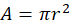. Here, topics like perimeter and area of a circle, areas of sector and segment of a circle as well as areas of combinations of plane figures will be discussed. On that note, Doubtnut provides that proves to be quite useful to the students in getting the right idea about it.

NCERT textbooks are important for the CBSE Students because NCERT books cover each and every topic as per the syllabus prescribed by cbse. It is seen that NCERT textbooks help students in clearing their basic concepts. But at the same time, students who wish to score good marks in the exams are not able to find success. This is because of the tricky questions that are set in the examinations which lead the students to get confused on how to attempt it in the best way. So, they feel disappointed for not being able to get good marks. For subjects like Math, if students fail to get the in-depth knowledge, it becomes difficult for them to obtain good marks in the exams. So, the only option that is left with them is to look forward to the different guide books that help them to clear all their queries.

With the aim to make the students get clear idea about the different concepts of the various subjects, a team of experienced tutors of Doubtnut have made their best effort to provide the right solutions. Here, they have prepared different useful online video tutorials which helps in clearing all the confusions in the best manner. Students can really find it much easier to get the perfect idea on how to attempt the different questions with the proper solutions. They also get to work on different exercises and examples that prove to be an added advantage to them. So, by following the step by step instructions provided by Doubtnut, it truly helps them to get all the right idea on how to attempt even the trickiest questions without any problem at all. You can find the best NCERT Class 10 Maths Solutions Chapter 12 in the right manner. Moreover, the NCERT solutions  provided in the form of videos and pdfs by doubtnut are useful for preparation of future competitive exams like IIT JEE, BITSAT etc.

Topics and subtopics included in NCERT Solutions for Class 10 Maths Chapter 12 Areas related to Circles

Introduction

In our daily life, we come across many objects that are related to the circular shape. Some of them are drain covers, cycle wheels, brooches, circular paths, etc. Thus, it is very important to find the perimeters and areas that are related to circular figures. Here, we will find out how to find the areas of different combinations of plane figures that involves circles or their parts.

Perimeter and Area of a Circle

You have learned that perimeter is the distance which is covered by traveling once around in a circle. A constant ration is beard by the circumference of a circle with its diameter. The Greek letter π(pie) denoted this constant ratio.

So, circumference = π

diameter

or, circumference = π x diameter

= π x2r (r being the radius of the circle)

= 2πr

We generally take the value of π as 22 or 3.14

7

Area of a circle is πr2. Let’s look at an example: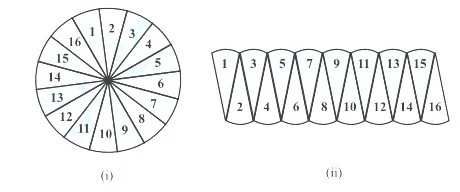Here, you can find that the figure (ii) has got a length ½ x 2πr along with the breadth r. So, here, the area of the circle = ½ x 2πr x r = πr2.  Let’s take another example to get the best idea about it.

In order to fence a circular field at the rate of Rs 24 per meter, the cost comes to Rs 5280. The field which needs to be ploughed should be at the rate of Rs 0.50 per m2. Now you need to find the cost of ploughing the field.

Solution: Length of the fence = Total cost = 5280 = 220

Rate         24

So, circumference of the field = 220m

If we take r metres as the radius of the field then,

2πr = 220

Or                            2 x 22 x r = 220

7

Or                            r = 220 x 7 = 35

2 x 22

i.e. radius of the field is 35m

So, area of the field =πr2 = 22 x 35 x 35,m2 = 22 x 5 x 35m2

7

Now the cost of ploughing 1m2 of the field= Rs 0.50

So, total cost of ploughing the field = Rs 22 x 5 x 35 x 0.50 = Rs 1925

Areas of Sector and Segment of a Circle

In your earlier classes, you have learned about sector and segment. Well, look at this figure below: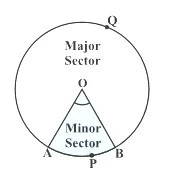Look at the shaded region OAPB. This happens to be a sector of the circle with centre O. The angle of the sector is AOB. OAPB is called the minor sector and OAQB is called the major sector. It can be clearly seen that the angle of the major sector is 360° – AOB.

Now look at another figure below: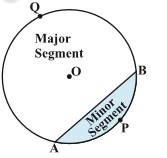Here, you can find that the shaded region APB is a segment of the circle.  So, APB is known as the minor segment while the major segment is AQB.

Now we will try to find some relations so as to make the calculation of their areas.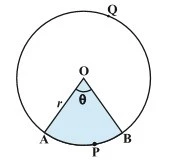Let OAPB be a sector of a circle where O and r are centre and radius respectively. We will assume that AOB be θ. So, as we know that area of a circle is πr2

So, it can be considered that this circular region is a sector that forms an angle of 3600. When we apply the Unitary Method, we get:

At 3600 measure of the angle, the area of the sector = πr2

When the centre is 1, the area of the sector = πr2

360

When the centre is 0, the area of the sector = πr2 x θ= θ x πr2

360       360

So, we obtain the following formula or relation for area of a sector of a circle:

Area of the sector of angle θ = θ x πr2

360

Here r and θ are the radius and angle respectively.

Areas of Combinations of Plane Figures

We have learned as to how it is possible to calculate the areas of different figures separately.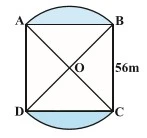In the figure above, there are two circular flower beds on two sides of a square lawn ABCD of side 56 m. You need to find the sum of the areas of the lawn and the flower beds with the point of intersection O.

Solution: Total area = Area of sector OAB + Area of sector ODC

+ Area of âˆ† OAD + Area of âˆ† OBC

= (90 x 22 x 28 x 56 x 90 x 22 x 28 x 56 + 1 x 56 x 56 + 1 x 56 x 56) m2

360   7                   360   7                    4                   4

= 1 x 28 x 56(22 + 22 +2 + 2) m2

4                 7      7

= 7 x 56 (22 + 22 + 14 + 14) m2

7

= 56 x 72 m2

= 4032 m2

Significance of Doubtnut for Class 10 students

Doubtnut’s online study materials prove to be useful to the students where they can attain the right knowledge on how to attempt different questions swiftly. Class 10 Maths NCERT Solutions is an added advantage to the students aspiring for competitive exams like JEE Mains, JEE Advanced, BITSAT etc. Some of the other advantages of learning math via Doubtnut are:

• Free of cost:  The students can access the study materials at free of cost and save their parent’s money.  So, the students do not have to look elsewhere as they can make the best use of the video tutorials which help in clearing their queries.
• Provides flexibility: Another best thing that the students enjoy is that they can download the study materials in the form of pdf. They can then try to access it no matter where they are. So, being very flexible, it is possible to find that it has proved to be of quite help in understanding the different topics easily.
• Expect the best support: Students can truly get the best support from Doubtnut which helps a lot to get rid of any sort of problems in attempting even the most difficult questions. This can lead them to scoring good marks in the exams without any worry. So, it is possible to be successful in exams by opting for the best and useful study materials provided by the expert tutors of Doubtnut.